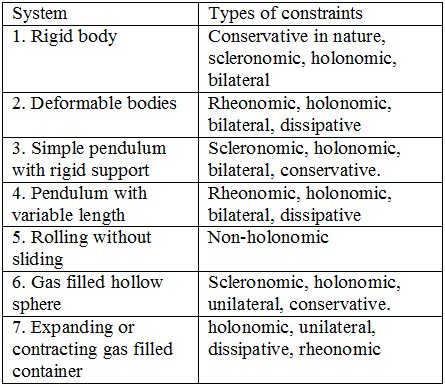# constraints in physics (classical mechanics) with examples

In this article learn about Constraints in physics used in classical mechanics. Constraints limit the motion of the system. We can use constraints to find the degrees of freedom that that must be calculated before solving dynamical problems.

## Constraints

• A constrained motion is a motion which cannot proceed arbitrarily in any manner.
• Particle motion can be restricted to occur (1) along with some specified path (2) on the surface (plane or curved) arbitrarily oriented in space.
• Imposing constraints on a mechanical system is done to simplify the mathematical description of the system.
• Constraints expressed in the form of equation $f(x_1,y_1,z_1,……,x_n,y_n,z_n :t)=0$ are called  holonomic constraints.
• Constraints not expressed in this fashion are called non-holonomic constraints.
• Scleronomic constraints are independent of time.
• Constraints containing time explicitly are called  rehonomic.

Therefore a constraint is either

Scleronomic where constraints relations does not depend on time or rheonomic where constraints relations depends explicitly on time

or

Holonomic where constraints relations can be made independent of velocity or non-holonomic where these relations are irreducible functions of velocity

Constraints types of some physical systems are given below in the tableSometimes motion of a particle or system of particles is restricted by one or more conditions. The limitations on the motion of the system are called constraints. The number of coordinates needed to specify the dynamical system becomes smaller when constraints are present in the system. Hence the degree of freedom of a dynamical system is defined as the minimum number of independent coordinates required to simplify the system completely along with the constraints. Thus if k is the number of constraints and N is the number of particles in the system possessing motion in three dimensions then the number of degrees of freedom are given by
n=3N-k                                  (1)
thus the above system has n degrees of freedom.
Constraints may be classified in many ways. If the condition of constraints can be expressed as equations connecting the coordinates of the particles and possibly the time having the form
f(r1,r2,……t)=0                          (2)
then constraints are said to be holonomic and the simplest example of holonomic constraints is a rigid body. In case of rigid body motion, the distance between any two particles of the body remains fixed and do not change with the tie. If ri and rj are the position vectors of the i’th and j’th particles then , the distance between the is given by
|ri-rj|=cij                                   (3)
The constraints which are not expressible in the form of equation 2 are called non-holonomic for example, the motion of a particle placed on the surface of a sphere of radius a will be described as
|r|≥a or, r-a≥0

Subscribe
Notify ofThis site uses Akismet to reduce spam. Learn how your comment data is processed.